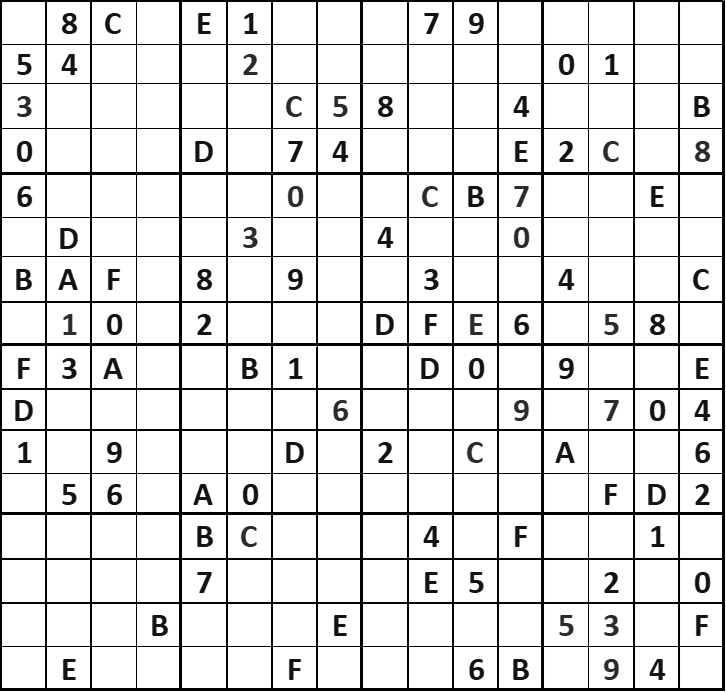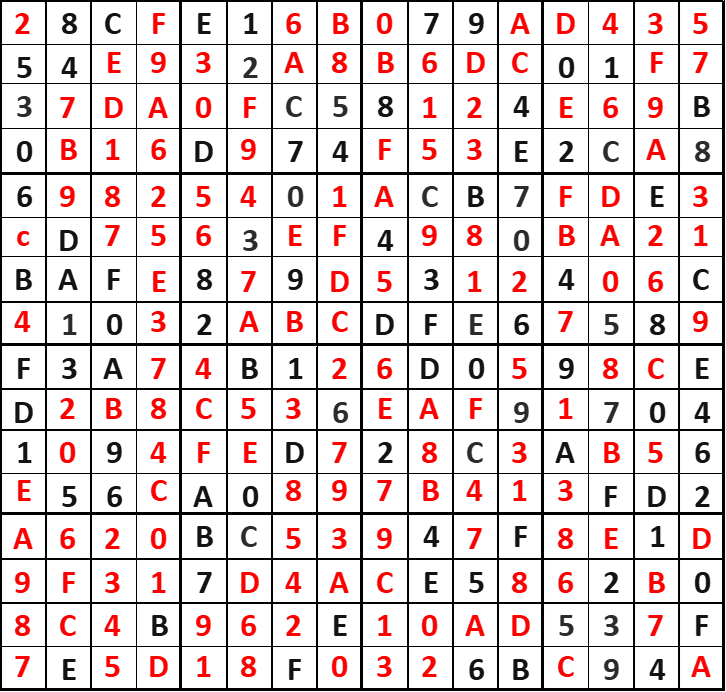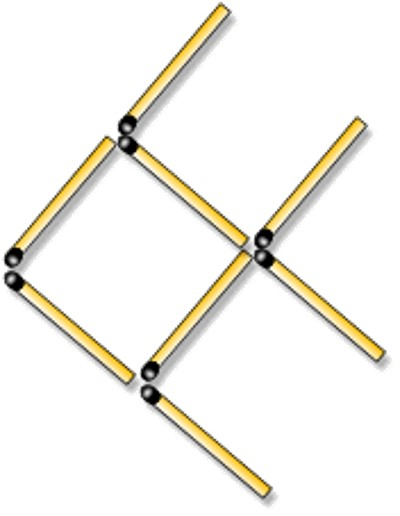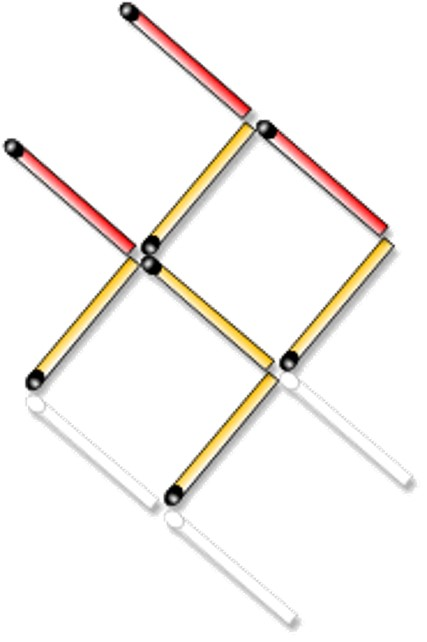Maths Challenge 8# Maths Challenge 8

## 1: Hexadoku Logic

This is a sudoku that uses the hexadecimal system of 16 characters (0,1,2,3,4,5,6,7,8,9,A,B,C,D,E,F).
The rules are the same as for a normal Sudoku, just with extra characters to include.Excel version of hexadoku grid

To get you started:
9th row down (beginning F3A); There is only one place the number 6 can go in this row.Excel verison of the completed hexadoku

## 2: How tall?Fractions

A boy wants to know how many of his class-mates are taller than him, and how many are smaller; so, after separating them into two groups, he finds that a quarter of the class are taller, two-thirds are smaller, and one boy is the same height.

How many boys are in his class?

What percentage is left over for the two boys of equal height?

1/4 + 2/3 = 3/12 + 8/12 = 11/12
This means 2 boys = 1/12 of the class
therefore the class has 2 x 12 = 24 boys.

## 3: Fairground oddsProbability (Higher GCSE/AS Level)

Ffion goes to a fair. There she finds a game that involves rolling 2 balls down an inclined plane so that each ball finally settles in one of five slots and scores the number of points allocated to that slot.

The slots are allocated the following values: 2, 4, 7, 4, 2.

It is possible for both balls to settle in the same slot.

It may be assumed that each slot is equally likely to accept either ball.

The player's score is the sum of the points scored by each ball.

If it costs 10p for each game and Ffion receives back a number of pence equal to her score, how much would Ffion expect to lose or gain in 50 games?

To start with, you will need to calculate the probabilities for each score and the mean average score.

Total Score Probability
4 4/25
6 8/25
8 4/25
9 4/25
11 4/25
14 1/25

Mean score = ((4 x 4) + (6 x 8) + (8 x 4) + (9 x 4) + (11 x 4) + (14 x 1)) ÷ 25 = 7.6

50 games at 10p each = £5.00
50 games winning average of 7.6p = £3.80
Total loss = £5.00 - £3.80 = £1.20

## 4: What's the number?Prime Numbers

What is the smallest prime number that is the sum of three different prime numbers?

One is not a prime number!

Prime numbers are: 2, 3, 5, 7, 11, 13, 17, 19, 23 ....
Cannot include the number 2 because this is the only even prime number meaning the answer will always be dividable by 2
So the answer is: 3 + 5 + 11 = 19

## 5: Swimming alongFun for All Ages

The below image shows a fish made from matchsticksThe challenge is to make the fish swim in the opposite direction by moving 3 of the matches

Using pencils, or some other object (not actual matchsticks please), recreate the image and use trial and error if necessary.